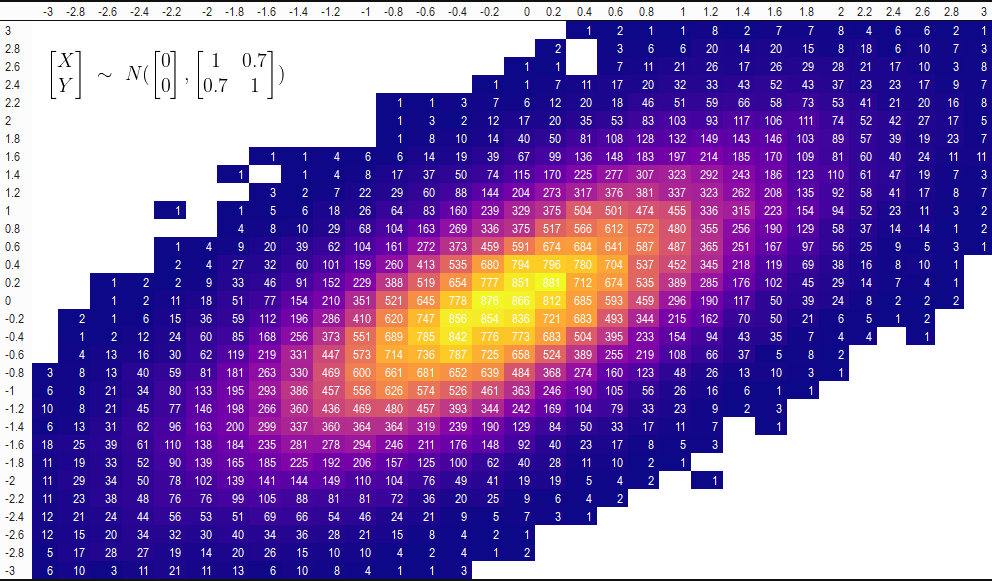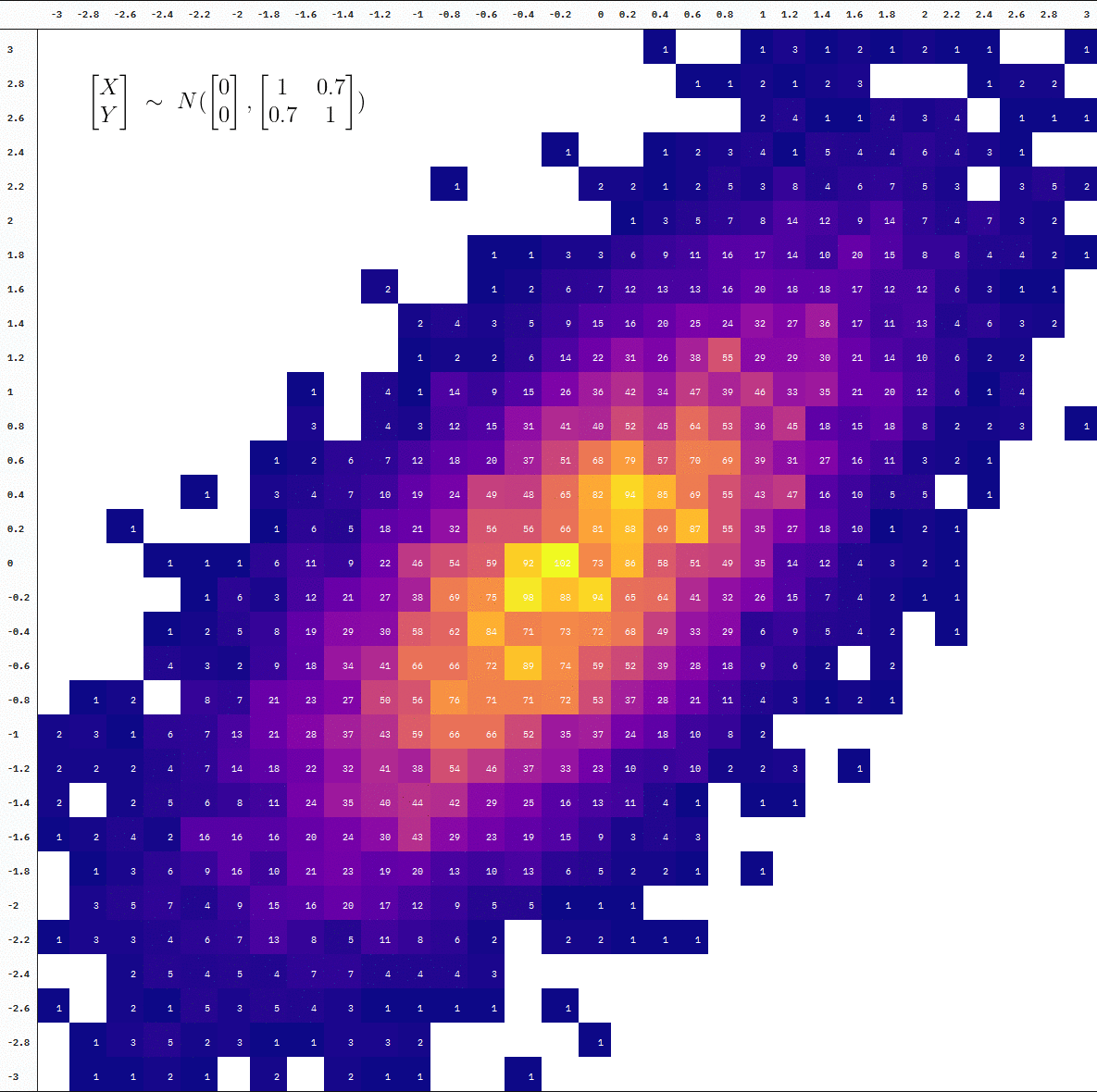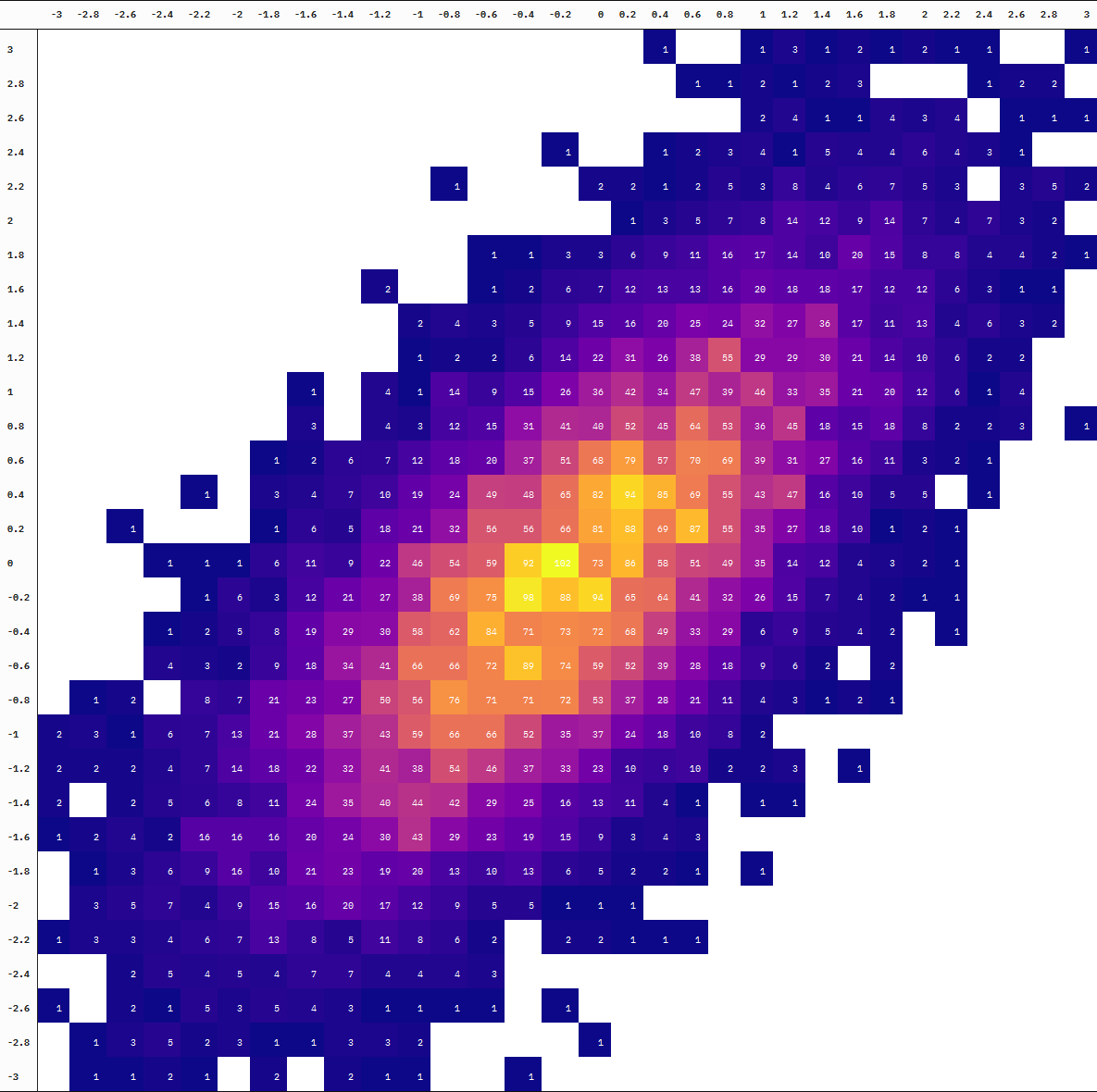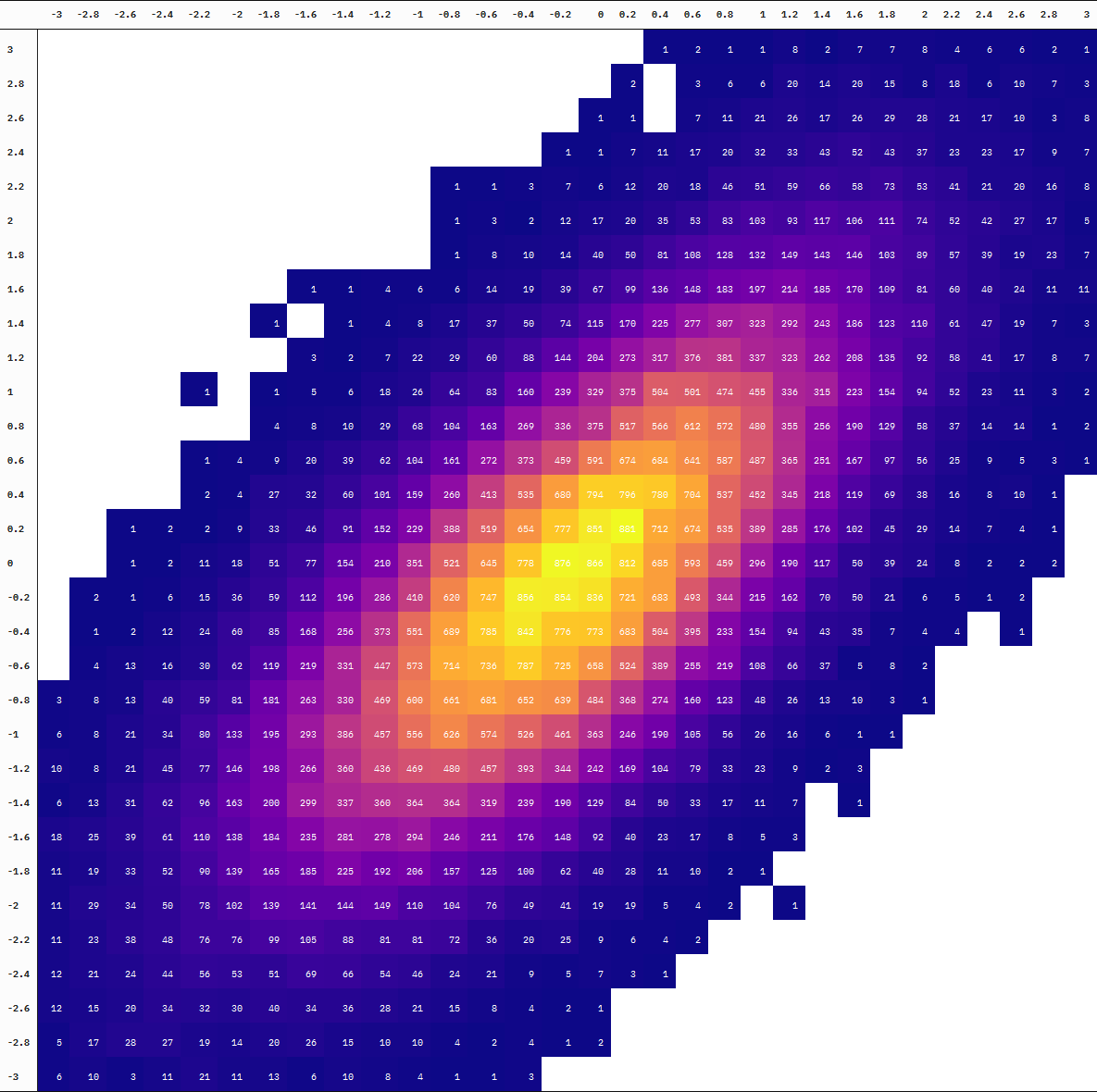# Random Sampling: A table animation

Plus a convenient way of rendering LaTeX expressions as images

June Choe (University of Pennsylvania Linguistics)https://live-sas-www-ling.pantheon.sas.upenn.edu/
01-17-2021

In my last blogpost, I demonstrated a couple use cases for the higher-order functionals reduce() and accumulate() from the {purrr} package. In one example, I made an animated {kableExtra} table by accumulate()-ing over multiple calls to column_spec() that set a background color for a column.

Animated tables are virtually non-existent in the wild, and probably for a good reason. but I wanted to extend upon my previous table animation and create something that’s maybe a bit more on the “informative” side.

To that end, here’s an animate table that simulates sampling from a bivariate normal distribution.

## Static

Let’s first start by generating 100,000 data points:

set.seed(2021)

library(dplyr)

samples_data <- MASS::mvrnorm(1e5, c(0, 0), matrix(c(1, .7, .7, 1), ncol = 2)) %>%
as_tibble(.name_repair = ~c("x", "y")) %>%
mutate(across(everything(), ~ as.character(.x - .x %% 0.2)))

samples_data

  # A tibble: 100,000 x 2
x     y
<chr> <chr>
1 0     -0.4
2 0.2   0.6
3 0.4   0.2
4 0.6   -0.2
5 0.6   0.8
6 -1.8  -2
7 0.8   -0.6
8 1.2   0.4
9 0.4   -0.4
10 1.4   1.6
# ... with 99,990 more rows

Let’s see how this looks when we turn this into a “matrix”1. To place continuous values into discrete cells in the table, I’m also binning both variables by 0.2:

samples_data_spread <- samples_data %>%
count(x, y) %>%
right_join(
tidyr::crossing(
x = as.character(seq(-3, 3, 0.2)),
y = as.character(seq(-3, 3, 0.2))
),
by = c("x", "y")
) %>%
tidyr::pivot_wider(names_from = y, values_from = n) %>%
arrange(-as.numeric(x)) %>%
select(c("x", as.character(seq(-3, 3, 0.2)))) %>%
rename(" " = x)


  # A tibble: 31 x 32
     -3 -2.8 -2.6 -2.4 -2.2  -2 -1.8 -1.6 -1.4 -1.2
<chr> <int>  <int>  <int>  <int>  <int> <int>  <int>  <int>  <int>  <int>
1 3        NA     NA     NA     NA     NA    NA     NA     NA     NA     NA
2 2.8      NA     NA     NA     NA     NA    NA     NA     NA     NA     NA
3 2.6      NA     NA     NA     NA     NA    NA     NA     NA     NA     NA
4 2.4      NA     NA     NA     NA     NA    NA     NA     NA     NA     NA
5 2.2      NA     NA     NA     NA     NA    NA     NA     NA     NA     NA
6 2        NA     NA     NA     NA     NA    NA     NA     NA     NA     NA
7 1.8      NA     NA     NA     NA     NA    NA     NA     NA     NA     NA
8 1.6      NA     NA     NA     NA     NA    NA     NA      1      1      4
9 1.4      NA     NA     NA     NA     NA    NA      1     NA      1      4
10 1.2      NA     NA     NA     NA     NA    NA     NA      3      2      7
# ... with 21 more rows, and 21 more variables: -1 <int>, -0.8 <int>,
#   -0.6 <int>, -0.4 <int>, -0.2 <int>, 0 <int>, 0.2 <int>,
#   0.4 <int>, 0.6 <int>, 0.8 <int>, 1 <int>, 1.2 <int>, 1.4 <int>,
#   1.6 <int>, 1.8 <int>, 2 <int>, 2.2 <int>, 2.4 <int>, 2.6 <int>,
#   2.8 <int>, 3 <int>

Now we can turn this into a table and fill the cells according to the counts using reduce():

library(kableExtra)

kable() %>%
kable_classic() %>%
column_spec(
kable_input = .x,
column = .y,
background = spec_color(
scale_from = c(1, max(as.numeric(as.matrix(samples_data_spread)), na.rm = TRUE)),
na_color = "white",
option = "plasma"
),
color = "white"
)},
.init = .
)

samples_data_table

-3 -2.8 -2.6 -2.4 -2.2 -2 -1.8 -1.6 -1.4 -1.2 -1 -0.8 -0.6 -0.4 -0.2 0 0.2 0.4 0.6 0.8 1 1.2 1.4 1.6 1.8 2 2.2 2.4 2.6 2.8 3
3 NA NA NA NA NA NA NA NA NA NA NA NA NA NA NA NA NA 1 2 1 1 8 2 7 7 8 4 6 6 2 1
2.8 NA NA NA NA NA NA NA NA NA NA NA NA NA NA NA NA 2 NA 3 6 6 20 14 20 15 8 18 6 10 7 3
2.6 NA NA NA NA NA NA NA NA NA NA NA NA NA NA NA 1 1 NA 7 11 21 26 17 26 29 28 21 17 10 3 8
2.4 NA NA NA NA NA NA NA NA NA NA NA NA NA NA 1 1 7 11 17 20 32 33 43 52 43 37 23 23 17 9 7
2.2 NA NA NA NA NA NA NA NA NA NA NA 1 1 3 7 6 12 20 18 46 51 59 66 58 73 53 41 21 20 16 8
2 NA NA NA NA NA NA NA NA NA NA NA 1 3 2 12 17 20 35 53 83 103 93 117 106 111 74 52 42 27 17 5
1.8 NA NA NA NA NA NA NA NA NA NA NA 1 8 10 14 40 50 81 108 128 132 149 143 146 103 89 57 39 19 23 7
1.6 NA NA NA NA NA NA NA 1 1 4 6 6 14 19 39 67 99 136 148 183 197 214 185 170 109 81 60 40 24 11 11
1.4 NA NA NA NA NA NA 1 NA 1 4 8 17 37 50 74 115 170 225 277 307 323 292 243 186 123 110 61 47 19 7 3
1.2 NA NA NA NA NA NA NA 3 2 7 22 29 60 88 144 204 273 317 376 381 337 323 262 208 135 92 58 41 17 8 7
1 NA NA NA NA 1 NA 1 5 6 18 26 64 83 160 239 329 375 504 501 474 455 336 315 223 154 94 52 23 11 3 2
0.8 NA NA NA NA NA NA 4 8 10 29 68 104 163 269 336 375 517 566 612 572 480 355 256 190 129 58 37 14 14 1 2
0.6 NA NA NA NA 1 4 9 20 39 62 104 161 272 373 459 591 674 684 641 587 487 365 251 167 97 56 25 9 5 3 1
0.4 NA NA NA NA 2 4 27 32 60 101 159 260 413 535 680 794 796 780 704 537 452 345 218 119 69 38 16 8 10 1 NA
0.2 NA NA 1 2 2 9 33 46 91 152 229 388 519 654 777 851 881 712 674 535 389 285 176 102 45 29 14 7 4 1 NA
0 NA NA 1 2 11 18 51 77 154 210 351 521 645 778 876 866 812 685 593 459 296 190 117 50 39 24 8 2 2 2 NA
-0.2 NA 2 1 6 15 36 59 112 196 286 410 620 747 856 854 836 721 683 493 344 215 162 70 50 21 6 5 1 2 NA NA
-0.4 NA 1 2 12 24 60 85 168 256 373 551 689 785 842 776 773 683 504 395 233 154 94 43 35 7 4 4 NA 1 NA NA
-0.6 NA 4 13 16 30 62 119 219 331 447 573 714 736 787 725 658 524 389 255 219 108 66 37 5 8 2 NA NA NA NA NA
-0.8 3 8 13 40 59 81 181 263 330 469 600 661 681 652 639 484 368 274 160 123 48 26 13 10 3 1 NA NA NA NA NA
-1 6 8 21 34 80 133 195 293 386 457 556 626 574 526 461 363 246 190 105 56 26 16 6 1 1 NA NA NA NA NA NA
-1.2 10 8 21 45 77 146 198 266 360 436 469 480 457 393 344 242 169 104 79 33 23 9 2 3 NA NA NA NA NA NA NA
-1.4 6 13 31 62 96 163 200 299 337 360 364 364 319 239 190 129 84 50 33 17 11 7 NA 1 NA NA NA NA NA NA NA
-1.6 18 25 39 61 110 138 184 235 281 278 294 246 211 176 148 92 40 23 17 8 5 3 NA NA NA NA NA NA NA NA NA
-1.8 11 19 33 52 90 139 165 185 225 192 206 157 125 100 62 40 28 11 10 2 1 NA NA NA NA NA NA NA NA NA NA
-2 11 29 34 50 78 102 139 141 144 149 110 104 76 49 41 19 19 5 4 2 NA 1 NA NA NA NA NA NA NA NA NA
-2.2 11 23 38 48 76 76 99 105 88 81 81 72 36 20 25 9 6 4 2 NA NA NA NA NA NA NA NA NA NA NA NA
-2.4 12 21 24 44 56 53 51 69 66 54 46 24 21 9 5 7 3 1 NA NA NA NA NA NA NA NA NA NA NA NA NA
-2.6 12 15 20 34 32 30 40 34 36 28 21 15 8 4 2 1 NA NA NA NA NA NA NA NA NA NA NA NA NA NA NA
-2.8 5 17 28 27 19 14 20 26 15 10 10 4 2 4 1 2 NA NA NA NA NA NA NA NA NA NA NA NA NA NA NA
-3 6 10 3 11 21 11 13 6 10 8 4 1 1 3 NA NA NA NA NA NA NA NA NA NA NA NA NA NA NA NA NA

### An aside on LaTeX equations

As an aside, let’s say we also want to annotate this table with the true distribution where this sample came from. As specified in our call to MASS::mvrnorm() used to make samples_data, the distribution is one where both variables have a mean of 0 and a standard deviation of 1, plus a correlation of 0.7:

$\begin{bmatrix} X \\ Y \end{bmatrix}\ \sim\ N(\begin{bmatrix} 0 \\ 0 \end{bmatrix},\begin{bmatrix}1 & 0.7 \\ 0.7 & 1 \end{bmatrix})$

Where the LaTeX code for the above formula is:

  \begin{bmatrix} X \\ Y \end{bmatrix}\ \sim\
N(\begin{bmatrix} 0 \\ 0 \end{bmatrix},
\begin{bmatrix}1 & 0.7 \\ 0.7 & 1 \end{bmatrix})

Many different solutions already exist to LaTeX math annotations. The most common is probably Non-Standard Evaluation (NSE) methods using parse(), expression(), bquote() etc. There are bulkier solutions like the {latex2exp} package that plots plotmath expressions, though it hasn’t been updated since 2015 and I personally had difficulty getting it to work.

One solution I’ve never heard of/considered before is querying a web LaTeX editor that has an API. The Online LaTeX Equation Editor by CodeCogs is the perfect example of this. A simple link that contains the LaTeX code in a URL-compatible encoding renders the resulting expression as an image!

I wrote a function latex_query (not thoroughly tested) in my personal package that takes LaTeX code and generates a CodeCogs URL containing the rendered expression2

# NOTE the string literal syntax using r"(...)" is only available in R 4.0.0 and up
latex_url <- junebug::latex_query(
formula = r"(\begin{bmatrix} X \\ Y \end{bmatrix}\ \sim\
N(\begin{bmatrix} 0 \\ 0 \end{bmatrix},
\begin{bmatrix}1 & 0.7 \\ 0.7 & 1 \end{bmatrix}))",
dpi = 150
)

knitr::include_graphics(latex_url)


The variable latex_url is this really long URL which, as we see above, points to a rendered image of the LaTeX expression we fed it!

Annotating our table, then, is pretty straightforward. We save it as an image, read in the LaTeX equation as an image, then combine!

save_kable(samples_data_table, "img/samples_data_table.png")

library(magick)

image_composite(
offset = "+50+50"
)## Animated

For an animated version, we add a step where we split the data at every 10,000 additional samples before binning the observations into cells. We then draw a table at each point of the accumulation using {kableExtra} with the help of map() and reduce() (plus some more kable styling).

samples_tables <- purrr::map(1L:10L, ~{
samples_slice <- samples_data %>%
slice(1L:(.x * 1e4)) %>%
count(x, y) %>%
right_join(
tidyr::crossing(
x = as.character(seq(-3, 3, 0.2)),
y = as.character(seq(-3, 3, 0.2))
),
by = c("x", "y")
) %>%
tidyr::pivot_wider(names_from = y, values_from = n) %>%
arrange(-as.numeric(x)) %>%
select(c("x", as.character(seq(-3, 3, 0.2)))) %>%
rename(" " = x)

samples_slice %>%
kable() %>%
kable_classic() %>%
purrr::reduce(
2L:length(samples_slice),
~ {
.x %>%
column_spec(
column = .y,
width_min = "35px",
background = spec_color(
samples_slice[[.y]],
scale_from = c(1, max(as.numeric(as.matrix(samples_slice)), na.rm = TRUE)),
na_color = "white",
option = "plasma"
),
color = "white"
) %>%
row_spec(
row = .y - 1L,
hline_after = FALSE,
)
},
.init = .
) %>%
row_spec(0L, bold = TRUE) %>%
column_spec(1L, bold = TRUE, border_right = TRUE) %>%
kable_styling(
full_width = F,
font_size = 10,
html_font = "IBM Plex Mono",
)
})


The result, samples_tables is a list of tables. We can walk() over that list with save_kable() to write them as images and then read them back in with {magick}:

purrr::iwalk(samples_tables, ~ save_kable(.x, file = glue::glue("tbl_imgs/tbl{.y}.png")))



Now we can add our LaTeX expression from the previous section as an annotation to these table images using image_composite():

table_imgs_annotated <- table_imgs %>%
image_composite(
offset = "+100+80"
)


Finally, we just patch the table images together into an animation using image_animate() and we have our animated table!

table_imgs_animated <- table_imgs_annotated %>%
image_animate(optimize = TRUE)


### Final ProductYou can also see the difference in the degree of “interpolation” by directly comparing the table at 10 thousand vs 100 thousand samples (the first and last frames):Neat!

1. Visually speaking. It’s still a dataframe object for compatibility with {kableExtra}↩︎

2. Details about the API - https://www.codecogs.com/latex/editor-api.php↩︎